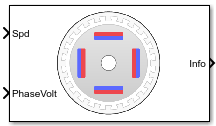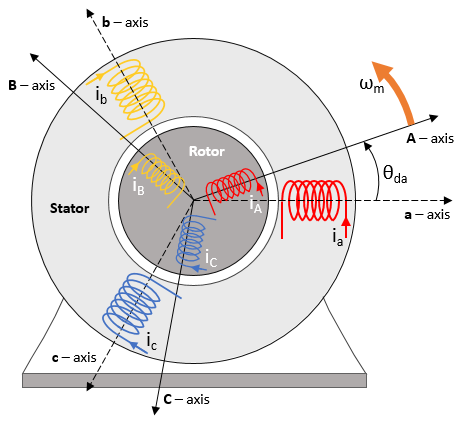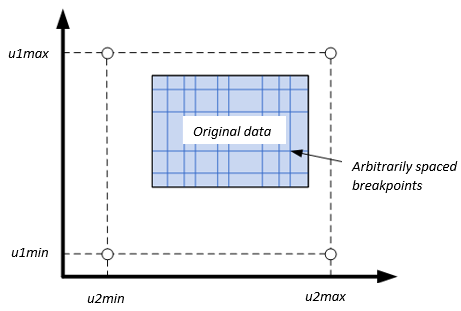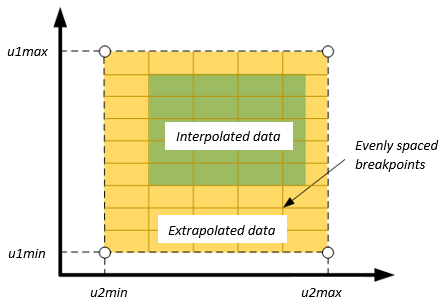Documentation

# Flux-Based PMSM

Flux-based permanent magnet synchronous motor

• Library:
• Powertrain Blockset / Propulsion / Electric Motors## Description

The Flux-Based PMSM block implements a flux-based three-phase permanent magnet synchronous motor (PMSM) with a tabular-based electromotive force. The block uses the three-phase input voltages to regulate the individual phase currents, allowing control of the motor torque or speed.

Flux-based motor models take into account magnetic saturation and iron losses. To calculate the magnetic saturation and iron loss, the Flux-Based PMSM block uses the inverse of the flux linkages. To obtain the block parameters, you can use finite-element analysis (FEA) or measure phase voltages using a dynamometer.

By default, the block sets the Simulation Type parameter to `Continuous` to use a continuous sample time during simulation. If you want to generate code for fixed-step double- and single-precision targets, considering setting the parameter to `Discrete`. Then specify a Sample Time, Ts parameter.

To enable power loss calculations suitable for code generation targets that limit memory, select Enable memory optimized 2D LUT.

### Three-Phase Sinusoidal Model Electrical System

The block implements equations that are expressed in a stationary rotor reference (dq) frame. The d-axis aligns with the a-axis. All quantities in the rotor reference frame are referred to the stator.The block uses these equations.

CalculationEquation
q- and d-axis voltage$\begin{array}{l}{v}_{d}=\frac{d{\psi }_{d}}{dt}+{R}_{s}{i}_{d}-{\omega }_{e}{\psi }_{q}\\ {v}_{q}=\frac{d{\psi }_{q}}{dt}+{R}_{s}{i}_{q}+{\omega }_{e}{\psi }_{d}\end{array}$
q- and d-axis current$\begin{array}{l}{i}_{d}=f\left({\psi }_{d},{\psi }_{q}\right)\\ {i}_{q}=g\left({\psi }_{d},{\psi }_{q}\right)\end{array}$
Electromechanical torque${T}_{e}=1.5P\left[{\psi }_{d}{i}_{q}-{\psi }_{q}{i}_{d}\right]$

The equations use these variables.

 ωm Rotor mechanical speed ωe Rotor electrical speed Θda dq stator electrical angle with respect to the rotor a-axis Rs, Rr Resistance of the stator and rotor windings, respectively iq, id q- and d-axis current, respectively vq, vd q- and d-axis voltage, respectively Ψq, Ψd q- and d-axis magnet flux, respectively P Number of pole pairs Te Electromagnetic torque

### Transforms

To calculate the voltages and currents in balanced three-phase (a, b) quantities, quadrature two-phase (α, β) quantities, and rotating (d, q) reference frames, the block uses the Clarke and Park Transforms.

In the transform equations.

TransformDescriptionEquations

Clarke

Converts balanced three-phase quantities (a, b) into balanced two-phase quadrature quantities (α, β).

Park

Converts balanced two-phase orthogonal stationary quantities (α, β) into an orthogonal rotating reference frame (d, q).

Inverse Clarke

Converts balanced two-phase quadrature quantities (α, β) into balanced three-phase quantities (a, b).

Inverse Park

Converts an orthogonal rotating reference frame (d, q) into balanced two-phase orthogonal stationary quantities (α, β).

The transforms use these variables.

 ωm Rotor mechanical speed P Motor pole pairs ωe Rotor electrical speed Θe Rotor electrical angle x Phase current or voltage

### Mechanical System

The rotor angular velocity is given by:

`$\begin{array}{c}\frac{d}{dt}{\omega }_{m}=\frac{1}{J}\left({T}_{e}-{T}_{f}-F{\omega }_{m}-{T}_{m}\right)\\ \frac{d{\theta }_{m}}{dt}={\omega }_{m}\end{array}$`

The equations use these variables.

 J Combined inertia of rotor and load F Combined viscous friction of rotor and load θm Rotor mechanical angular position Tm Rotor shaft torque Te Electromagnetic torque Tf Combined rotor and load friction torque ωm Rotor mechanical speed

### Power Accounting

For the power accounting, the block implements these equations.

Bus Signal DescriptionVariableEquations

`PwrInfo`

`PwrTrnsfrd` — Power transferred between blocks

• Positive signals indicate flow into block

• Negative signals indicate flow out of block

`PwrMtr`

Mechanical power

Pmot

`PwrBus`

Electrical power

Pbus

`PwrNotTrnsfrd` — Power crossing the block boundary, but not transferred

• Positive signals indicate an input

• Negative signals indicate a loss

`PwrElecLoss`

Resistive power loss

Pelec

`PwrMechLoss`

Mechanical power loss

Pmech

When Port Configuration is set to `Torque`:

When Port Configuration is set to `Speed`:

`PwrStored` — Stored energy rate of change

• Positive signals indicate an increase

• Negative signals indicate a decrease

`PwrMtrStored`

Stored motor power

Pstr

The equations use these variables.

 Rs Stator resistance ia, ib, ic Stator phase a, b, and c current isq, isd Stator q- and d-axis currents van, vbn, vcn Stator phase a, b, and c voltage ωm Angular mechanical velocity of the rotor F Combined motor and load viscous damping Te Electromagnetic torque Tf Combined motor and load friction torque

### Lookup Table Memory Optimization

The data for the Corresponding d-axis current, id and Corresponding q-axis current, iq lookup tables are functions of the d- and q-axis flux.

To enable current calculations suitable for code generation targets that limit memory, select Enable memory optimized 2D LUT. The block uses linear interpolation to optimize the current lookup table values for code generation. This table summarizes the optimization implementation.

Use CaseImplementation

d- and q-axis flux aligns with the lookup table breakpoint values.

Memory-optimized current is current lookup table value at intersection of flux values.

d- and q-axis flux does not align with the lookup table breakpoint values, but is within range.

Memory-optimized current is linear interpolation between corresponding flux values.

d- and q-axis flux does not align with the lookup table breakpoint values, and is out of range.

Cannot compute an memory-optimized current. Block uses extrapolated data.

#### Extrapolation

The lookup tables optimized for code generation do not support extrapolation for data that is out of range. However, you can include pre-calculated extrapolation values in the power loss lookup table by selecting Specify Extrapolation.

The block uses the endpoint parameters to resize the table data.

User InputExtrapolation## Ports

### Input

expand all

Rotor shaft input torque, Tm, in N·m.

#### Dependencies

To create this port, select `Torque` for the Port Configuration parameter.

Angular velocity of the rotor, ωm, in rad/s.

#### Dependencies

To create this port, select `Speed` for the Port Configuration parameter.

Stator terminal voltages, Va, Vb, and Vc, in V.

#### Dependencies

To create this port, select `Speed` or `Torque` for the Port Configuration parameter.

### Output

expand all

The bus signal contains these block calculations.

Signal DescriptionVariableUnits

`IaStator`

Stator phase current A

ia

A

`IbStator`

Stator phase current B

ib

A

`IcStator`

Stator phase current C

ic

A

`IdSync`

Direct axis current

id

A

`IqSync`

iq

A

`VdSync`

Direct axis voltage

vd

V

`VqSync`

vq

V

`MtrSpd`

Angular mechanical velocity of the rotor

ωm

`MtrPos`

Rotor mechanical angular position

θm

`MtrTrq`

Electromagnetic torque

Te

N·m

`PwrInfo`

`PwrTrnsfrd`

`PwrMtr`

Mechanical power

Pmot

W
`PwrBus`

Electrical power

Pbus

W

`PwrNotTrnsfrd`

`PwrElecLoss`

Resistive power loss

Pelec

W
`PwrMechLoss`

Mechanical power loss

Pmech

W
`PwrStored``PwrMtrStored`

Stored motor power

Pstr

W

Phase a, b, c current, ia, ib, and ic, in A.

Motor torque, Tmtr, in N·m.

#### Dependencies

To create this port, select `Speed` for the Port configuration parameter.

Angular speed of the motor, ωmtr, in rad/s.

#### Dependencies

To create this port, select `Torque` for the Port configuration parameter.

## Parameters

expand all

#### Block Options

By default, the block uses a continuous sample time during simulation. If you want to generate code for single-precision targets, considering setting the parameter to `Discrete`.

#### Dependencies

Setting Simulation Type to `Discrete` creates the Sample Time, Ts parameter.

Integration sample time for discrete simulation, in s.

#### Dependencies

Setting Simulation Type to `Discrete` creates the Sample Time, Ts parameter.

This table summarizes the port configurations.

Port ConfigurationCreates Input PortCreates Output Port

`Torque`

`LdTrq`

`MtrSpd`

`Speed`

`Spd`

`MtrTrq`

Enable generation of optimized lookup tables, suitable code generation targets that limit memory.

d-axis flux, Ψd, breakpoints, in Wb.

Flux breakpoint storage size, n1, dimensionless. The block resamples the Corresponding d-axis current, id and Corresponding q-axis current, iq data based on the storage size.

#### Dependencies

To create this parameter, select Enable memory optimized 2D LUT.

q-axis flux, Ψq, breakpoints, in Wb.

Flux breakpoint storage size, n2, dimensionless. The block resamples the Corresponding d-axis current, id and Corresponding q-axis current, iq data based on the storage size.

#### Dependencies

To create this parameter, select Enable memory optimized 2D LUT.

Array of values for d-axis current, id, as a function of `M` d-fluxes, Ψd, and `N` q-fluxes, Ψq, in A. Each value specifies the current for a specific combination of d- and q-axis flux. The array size must match the dimensions defined by the flux vectors.

If you set Enable memory optimized 2D LUT, the block converts the data to single precision.

Array of values for q-axis current, id, as a function of `M` d-fluxes, Ψd, and `N` q-fluxes, Ψq, in A. Each value specifies the current for a specific combination of d- and q-axis flux. The array size must match the dimensions defined by the flux vectors.

If you set Enable memory optimized 2D LUT, the block converts the data to single precision.

Flux breakpoint maximum extrapolation endpoint, u1max, in Wb.

#### Dependencies

To create this parameter, select Enable memory optimized 2D LUT and Specify Extrapolation.

Flux breakpoint minimum extrapolation endpoint, u1min, in Wb.

#### Dependencies

To create this parameter, select Enable memory optimized 2D LUT and Specify Extrapolation.

Flux breakpoint maximum extrapolation endpoint, u2max, in Wb.

#### Dependencies

To create this parameter, select Enable memory optimized 2D LUT and Specify Extrapolation.

Flux breakpoint minimum extrapolation endpoint, u2min, in Wb.

#### Dependencies

To create this parameter, select Enable memory optimized 2D LUT and Specify Extrapolation.

Stator phase resistance, Rs, in ohm.

Motor pole pairs, P.

Initial d- and q-axis flux, Ψq0 and Ψd0, in Wb.

Initial rotor angular position, θm0, in rad.

Initial angular velocity of the rotor, ωm0, in rad/s.

#### Dependencies

To enable this parameter, select the `Torque` configuration parameter.

Mechanical properties of the rotor:

• Inertia, J, in kgm^2

• Viscous damping, F, in N·m/(rad/s)

• Static friction, Tf, in N·m

#### Dependencies

To enable this parameter, select the `Torque` configuration parameter.

 Hu, Dakai, Yazan Alsmadi, and Longya Xu. “High fidelity nonlinear IPM modeling based on measured stator winding flux linkage.” IEEE® Transactions on Industry Applications, Vol. 51, No. 4, July/August 2015.

 Chen, Xiao, Jiabin Wang, Bhaskar Sen, Panagiotis Lasari, Tianfu Sun. “A High-Fidelity and Computationally Efficient Model for Interior Permanent-Magnet Machines Considering the Magnetic Saturation, Spatial Harmonics, and Iron Loss Effect.” IEEE Transactions on Industrial Electronics, Vol. 62, No. 7, July 2015.

 Ottosson, J., M. Alakula. “A compact field weakening controller implementation.” International Symposium on Power Electronics, Electrical Drives, Automation and Motion, July, 2006.

##### Support평가판 신청